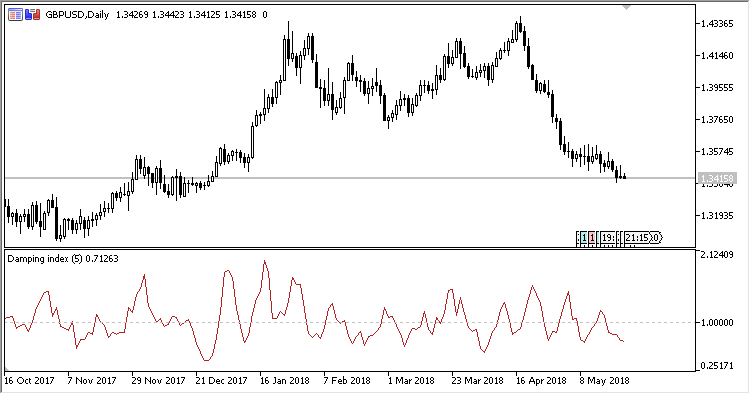# DI – indicator for MetaTrader 5

Oscillator DI (Damping Index) is designed for identifying the damping of the directed market movement.

Any value below 1.0 definitely points to slowing price movement, whatever direction it was moving before.

It has three input parameters:

• Period – calculation period;
• Method – calculation method;
• Damping level – threshold level.
Related Posts

Calculations:

`DI = (H - L)/R`

where:

```H = Moving Average(High price, Period, Method)
L = Moving Average(Low price, Period, Method)
R = H[i-Period] - L[i-Period]
```